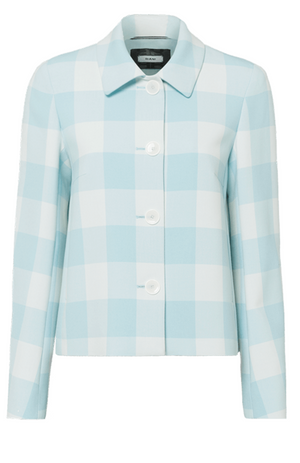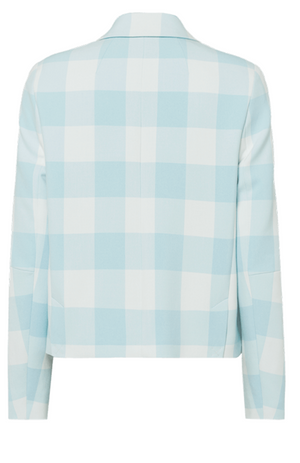Riani

# Blazer

Regular price \$795 now \$397.50 Unit price  per

Shipping calculated at checkout.
• 68% polyester 28% viscose 4% elasthan
• Dry clean only
• Boxy fit
• Great rendition of a classic
• Chest darts & dividing seams
• 60s vibe

Size Guide

• 34 = 4 = XS
• 36 = 6 = S
• 38 = 8 = M
• 40 = 10 = M
• 42 = 12 = L
• 44 = 14 = L
• 46 = 16 = XL